# 为什么我要放弃javaScript数据结构与算法（第三章）—— 栈

+关注继续查看

## 第三章 栈

### 创建栈

1. 先声明这个类
   function Stack(){
// 各种属性和方法的声明
}
1. 选择数组这种数据结构来保存栈里的元素
   let items = [];
1. 为栈声明一些方法

• push（element（s））： 添加一个（或者几个）新元素到栈顶
• pop（）：移除栈顶的元素，同时返回被移除的元素
• peek（）：返回栈顶的元素，不会对栈做任何修改（这个方法不会移除栈顶的元素，仅仅返回它）
• isEmpty（）：如果栈里没有任何元素的就返回true，否则就返回false.
• clear（）：移除栈里的所有元素
• size（）：返回栈里的元素个数，这个方法和数组的length属性很类似。

### 向栈添加元素

this.push = function(element){
return items.push(element);
}

this.pop = function(){
return items.pop();
}

### 查看栈顶元素

this.peek = function(){
return items[items.length-1];
}

isEmpty ,如果栈为空的话就返回true，否则就返回false

this.isEmpty = function(){
return items.length == 0;
}

this.size = function(){
return items.length;
}

### 清空和打印栈元素

this.clear = function(){
items = [];
return null;
}

this.print = function(){
console.log(items.toString());
}

### 实例

function Stack(){
let items = [];
this.push = function(element){
return items.push(element);
}
this.pop = function(){
return items.pop();
}
this.peek = function(){
return items[items.length-1];
}
this.isEmpty = function(){
return items.length == 0;
}
this.size = function(){
return items.length;
}
this.clear = function(){
items = [];
}
this.print = function(){
console.log(items.toString());
}
}
let stack = new Stack();
console.log(stack.isEmpty()); // true 判断是否为空
stack.push(5); // 往栈里添加元素 5
stack.push(8); // 往栈里添加元素 8
console.log(stack.peek()); // 查看最后一个元素 8
stack.push(11); // 往栈里添加元素 11
console.log(stack.size()); // 3 输出栈的元素个数
console.log(stack.isEmpty()); // false 判断是否为空
stack.push(15); // 往栈里添加元素 15
stack.print(); // 5,8,11,15 输出栈里的元素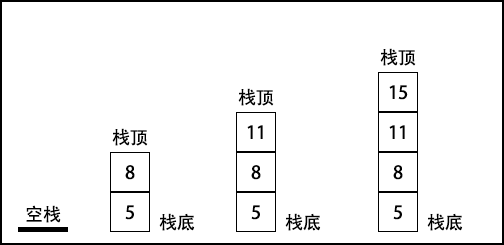### 用 ES6 声明 Stack 类

class Stack{
constructor(){
this.items = []; // {1}
}
push(elememt){
this.items.push(element);
}
// 其他方法
}

#### 用ES6的限定作用域 Symbol 实现类

ES6 新增了一种叫做 Symbol 的基本类型，它是不可变的，可以用作对象的属性。

let _items = Symbol(); // 声明了 Symbol 类型的变量
class Stack{
constructor(){
this[_items] = [] // 要访问 _items,只需把所有的 this.items都换成 this.[_items]
}
push(element){
return this[_items].push(element);
}
pop (){
return this[_items].pop();
}
peek (){
return this[_items][this[_items].length-1];
}
isEmpty (){
return this[_items].length == 0;
}
size (){
return this[_items].length;
}
clear (){
this[_items] = [];
}
print (){
console.log(this[_items].toString());
}
}

let stack = new Stack();
stack.push(5);
stack.push(8);
let objectSymbols = Object.getOwnPropertySymbols(stack);
console.log(objectSymbols.length); // 1
console.log(objectSymbols); // [Symbol()]
console.log(objectSymbols); // Symbol()
stack[objectSymbols].push(1);
stack.print(); // 5,8,1

#### 用ES6类的 WeakMap 实现类

const items = new WeakMap(); // 声明了一个 WeakMap 类型的变量 items
class Stack{
constructor(){
items.set(this, []) // 在 constructor 中，以this（Stack类自己引用）为键，把代表栈的数组存入 items
}
push(element){
let s = items.get(this);
s.push(element);
}
pop (){
let s = items.get(this);
let r = s.pop();
return r;
}
peek (){
let s = items.get(this);
return s[s.length-1];
}
isEmpty (){
let s = items.get(this);
return s.length == 0;
}
size (){
let s = items.get(this);
let r = s.length
return r;
}
clear (){
items.set(this, [])
}
print (){
let s = items.get(this);
console.log(s.toString());
}
}

let stack = (function(){
const items = new WeakMap();
class Stack {
constructor(){
items.set(this, []);
}
// 其他方法
}
return Stack; // 当 Stack 函数里的构造函数被调用时，会返回 Stack 类的一个实例。
})()

### 用栈解决问题

#### 从十进制到二进制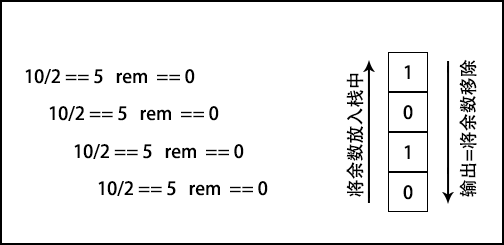function divideBy2(decNumber){
var remStack = new Stack(),
rem,
binaryString = '';
while(decNumber > 0){
rem = Math.floor(decNumber % 2); // 拿到被2整除的余数
remStack.push(rem);
decNumber = Math.floor(decNumber / 2) // 拿到被2整除的整数
}
while (! remStack.isEmpty()){
binaryString += remStack.pop().toString();
}
return binaryString;
}

console.log(divideBy2(10)); // 1010
console.log(divideBy2(233)); // 11101001
console.log(divideBy2(100)); // 11101001

JavaScript有数字类型，但是不会区分究竟是整数还是浮点数，使用 Math.floor 让除法只返回整数部分。

### 进制转换算法

function baseConverter(decNumber, base){
var remStack = new Stack(),
rem,
baseString = '',
digits = '0123456789ABCDEF';
while(decNumber > 0){
rem = Math.floor(decNumber % base); // 拿到被base整除的余数
remStack.push(rem);
decNumber = Math.floor(decNumber / base) // 拿到被base整除的整数
}
while (! remStack.isEmpty()){
baseString += digits[remStack.pop()];
}
return baseString;
}
console.log(baseConverter(100345,2)); // 11000011111111001
console.log(baseConverter(100345, 8)); // 303771
console.log(baseConverter(100345, 16)); // 187F9

### 小结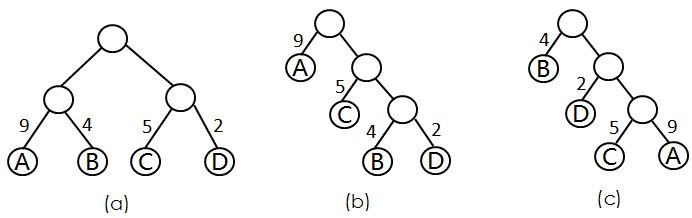4 0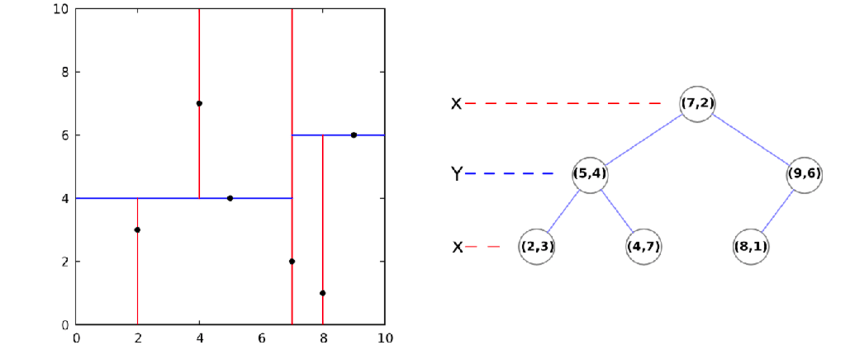10 0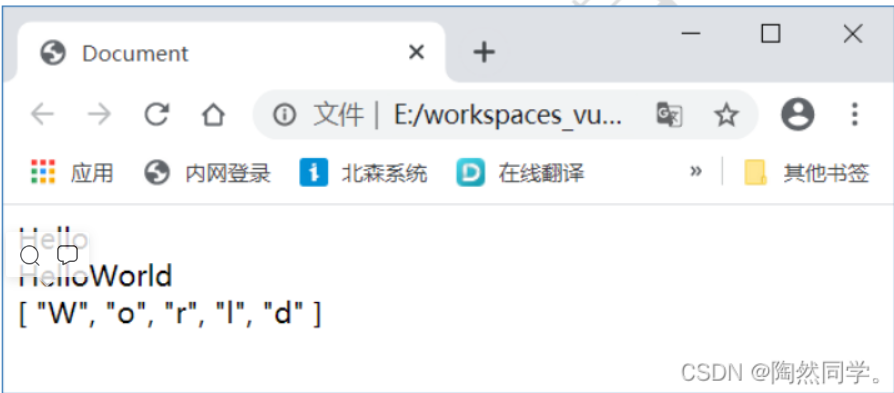Vue入门 基本使用 与 事务管理【1】
Vue入门 基本使用 与 事务管理【1】
9 04 0Java - 集合容器
Java - 集合容器
4 0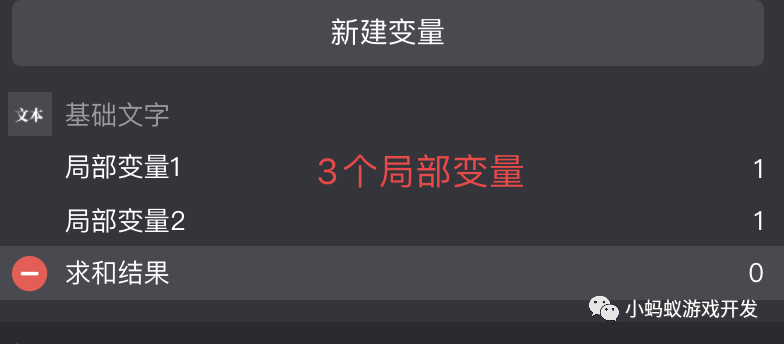7 04 0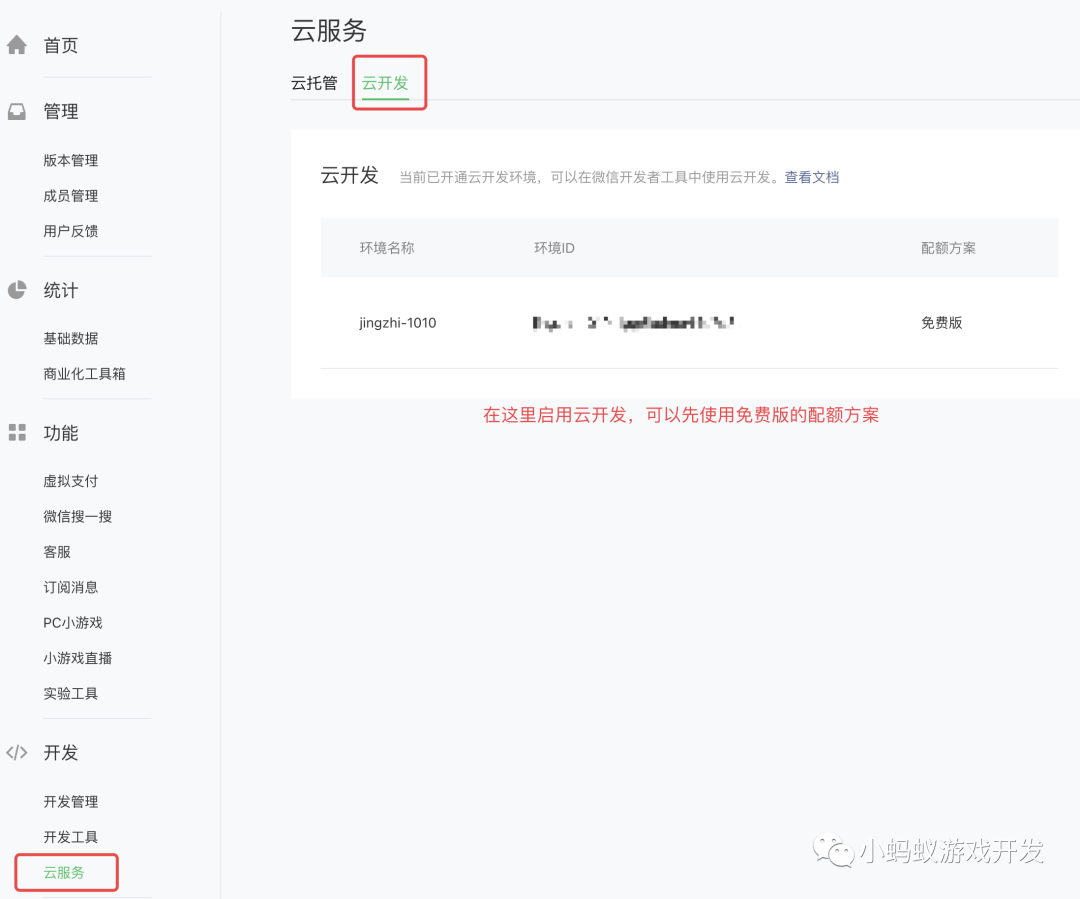9 0

11

0

OceanBase 入门到实战教程